American Journal of Civil Engineering
Volume 4, Issue 5, September 2016, Pages: 247-253

Parametric Study of the Lateral Behavior of Cast in Drilled Hole Piles

Samir Abdul Baki Jabbar Al-Jassim1, Rafi Mohammed Qasim2

1Department of Civil Engineering, University of Basra, Basra, Iraq

2Department of Environment and Pollution Engineering, Basra Engineering Technical College, Southern Technical University, Basra, Iraq(S. A. B. J. Al-Jassim)

Samir Abdul Baki Jabbar Al-Jassim, Rafi Mohammed Qasim. Parametric Study of the Lateral Behavior of Cast in Drilled Hole Piles. American Journal of Civil Engineering. Vol. 4, No. 5, 2016, pp. 247-253. doi: 10.11648/j.ajce.20160405.16

Received: July 8, 2016; Accepted: July 18, 2016; Published:August 15, 2016

Abstract: A cast in drilled hole (CIDH) piles are to be used as a bridge foundation. The geotechnical data of the site indicate that the soil profile consist of 10m normally consolidated soft clay underlined by a thick layer of over consolidated stiff clay. Three different pile diameters of 1.6m, 1.8m and 2m are selected from the analysis to be used for a depth of 30m below ground level and an average height of 5m above ground level. To investigate behavior of these (CIDH) piles under lateral loads, an analytical parametric study is performed to evaluate the ultimate lateral load capacity of the piles (which is assumed to cause a pile head displacement of 10% of the pile diameter) and the distribution of shear force and bending moment along the depth of the piles. The soil is represented by two ways, linear and nonlinear material. For the linear case, a linear brick finite element is used to represent the soil with either a linearly variable modulus of elasticity from ground level to the bottom of the pile or a constant modulus of elasticity for the top 10 meters (the soft clay) while linearly varying for the next 20m. For the nonlinear case, the P-Y curves method is used to represent the soil by nonlinear springs at intervals of 1 meter. In both cases (linear and nonlinear soil), the piles are assumed to behave linearly. Results obtained indicate that the ultimate lateral load capacity of the piles from the nonlinear case is in the range of 50% to 60% of the linear case.

Keywords: Cast in Drilled Hole Piles, P-Y Curves, Finite Element

1. Introduction

In this paper, two methods (P-Y curve method and elastic finite element method) are employed to analyze a laterally loaded circular (CIDH) piles. The piles are embedded 30meters in clayey soil and extended to an average of 5m above ground level. The clay deposit consists of two layers; the top 10m is normally consolidated soft clay while the underlined layer which has a depth of 30m is over consolidated stiff clay. Comparison between the results of the two methods has been done.

2. Problem Definition and Objective

A cast in drilled hole piles are intended to be used as a bridge foundation. The geotechnical data of the site show that the soil profile consists of a top layer of normally consolidated soft clay up to a depth of 10meters below ground level. This layer is underlined by a thick layer of over consolidated stiff clay which extends to a depth of 40m below ground level. Soil properties are given in table 1. A preliminary design data resulted in selecting 3 different piles diameters to be used 1.6m, 1.8m and 2m according to the load transferred from the deck to the pile. It is required to investigate: (1)- The ultimate lateral load capacity of the piles. (2)- The shear force and bending moment distribution along the length of the piles. The piles length selected to be 30m below ground level and an average of 5m above ground level.

Table 1. Site soil properties.

 Layer Depth (m) Modulus of elasticity –E MPa Poisson's ratio Bulk density KN/m3 Unconfined shear strength KPa 1 0 - 10 6 in average 0.4 17 20 2 10 – 40 Linearly varying from 14 to 36 0.4 18 50 - 110

3. Numerical Modeling

Two methods are employed to model and analyze the piles, namely the P-Y curve method and the elastic continuum finite element method.

3.1. The P-Y Curve Method

In this method, the piles are modeled as linear elastic two nodes frame element with circular section and six degrees of freedom at each node. The Young's modulus, Poisson's ratio and unit weight for all the piles are equal 25.75MPa, 0.25 and 24KN/m3 respectively. The soil is modeled as nonlinear springs spacing at 1 meter interval. The stiffness of the springs are evaluated from P-Y curves for soft clay (Matlock, 1970)  for the first 10 meters and from the P-Y curves for stiff clay (Reese et al., 1975)  for the lower 20 meter of the piles. The lateral load is applied at the pile head (5m above ground) and the pile head assumed to undergo only translational displacements (no rotation allowed). ANSYS 12.1 program is used to solve the problem.

3.2. The Elastic Continuum Finite Element Method

In this method, the piles are modeled as before with the same type of element and properties. The soil domain is modeled by 8 node linear brick element with only translational degrees of freedom. The elastic modulus of soil and Poisson's ratio are the only factors required for the analysis. The Poisson's ratio is assumed constant and equal 0.4 while the elastic modulus of soil is represented in two different ways:

(a)– It is assumed to vary linearly with the depth from 5 MPa at the ground level to 36 MPa at 40 meter below ground level then remain constant (36 MPa) for the remaining depth.

(b)– The top 10 meters of the soil is assumed to have a constant elastic modulus of 6 MPa (as suggested by Reese and Matlock, 1956; Poulos and Davis, 1980) [27, 22], while the elastic modulus for the second layer is varying linearly from 14 MPa at 10 meter below ground level to 36 MPa at a depth of 40 meter below ground level. The remaining depth of soil is assumed to have constant modulus of 36 MPa.

The soil domain used to model the problem is extended to 10 pile diameter on each side and below the pile (Robert Cook, 1995) . The boundary conditions applied at the ends of soil domain is pinned (no translation) in all directions except the top surface of the soil is kept free. The connection between the pile and soil is taken as perfect bond.

In both methods (the P-Y curve method and the finite element method) the control factor in evaluating the ultimate lateral load capacity of the piles is the yield of the maximum allowed lateral displacement at the pile head which is taken to be 10% of the pile diameter as it is more compliant with the design criterion (ASTM STP-835, 1983 and USACE, 1998) [17, 37].

4. Results and Discussions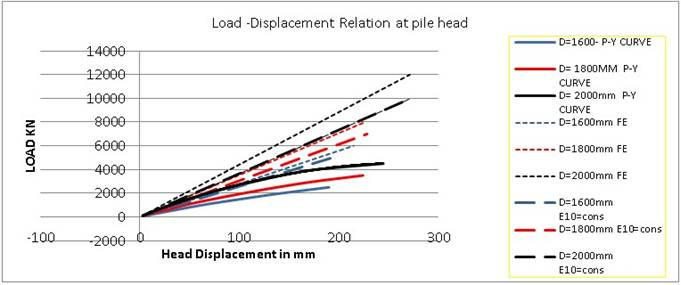The variation of the piles head displacement due to the loads applied at the piles head are drawn in figure 1. It can be seen from the figure that the variation of the pile head displacement with the applied loads are nonlinear for the case of P-Y curve method, while it is linear for both cases of the finite element method. This result is expected as the soil is assumed completely linear material in the finite element cases.

4.2. Ultimate Lateral Load Capacity of Piles

In all the cases studied, the load required to displace the pile head (5m above ground level) a horizontal distance equal to 10% of the pile diameter is assumed as the ultimate load capacity of the pile. These ultimate loads are listed in table 2. It can be seen that the ultimate load capacity of the piles from the linear finite element analysis in general is greater than that of the P-Y curve method (from 70% to 75% greater for the case of a constant top soil properties and from 95% to 100% grater for the case of linearly varying soil properties). This difference is because of the P-Y curve method is usually underestimate the soil resistance and do not consider the effect of the fixity conditions at the pile head (Dewaikar et al., 2009; Wallace et al., 2014) [12, 39]. In contrast the linear finite element method is over estimate the soil resistance due to the assumption of a linear soil behavior which is actually not correct especially at the top soil layer, and also the assumption of a perfect bond between the soil and the pile makes the soil respond equally to the displacement of the pile in tension and compression which is actually different.

Table 2. Lateral ultimate load capacity of piles.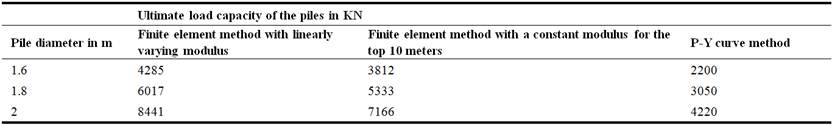Due to the above discussion, the actual ultimate lateral load capacity of the piles is expected to be in between the two results (the P-Y curve method result and the finite element method result). However due to the high uncertainties in predicting the soil properties in the site, the results of the P-Y curve method are assumed acceptable however they are conservative.

4.3. The Deformed Shape of the Piles Under the Ultimate Loads

The deformed shapes of the piles under ultimate lateral loads are shown in figure 2. A good matching of the deformed shapes from the three cases is obtained. However it is evident that the deformation is higher from the case of P-Y curve method. Also it is clearly visible from figure 2 that the extension of the deformation to the bottom of the pile is directly proportional to the pile rigidity (represented by pile diameter since all the piles have the same material). The effect of pile rigidity is more visible from the toe displacement as shown in figure 2c.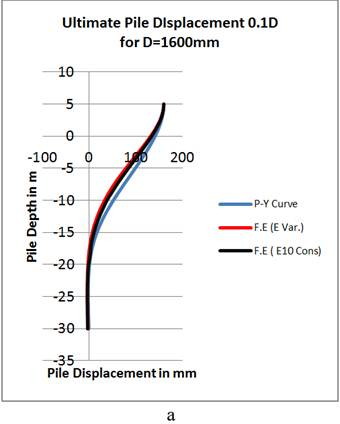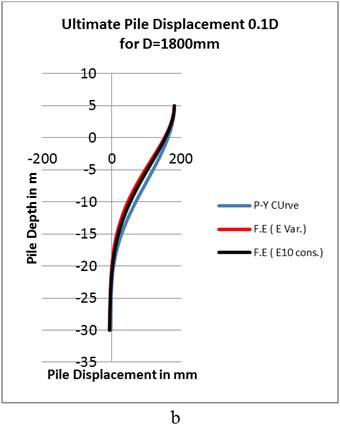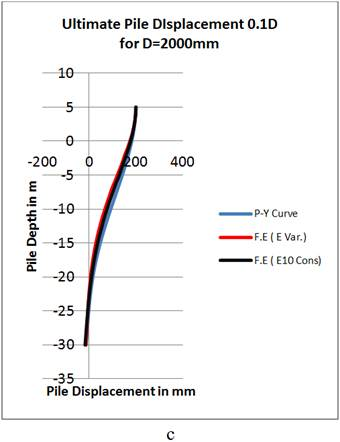Figure 2. The deformed shapes of the piles under ultimate loads. (a- 1.6m diameter piles, b- 1.8m diameter piles and c- 2m diameter piles).

4.4. The Variation of Shear Force and Bending Moment Along the Length of the Piles

The variations of the shear force along the length of the pile are given in figure 3. It is clearly visible the difference of the shear force values (due to different loads applied) and shear force distribution (due to different simulation of the soil) between the P-Y curve method and the finite element method. Again the effect of the pile rigidity is reflected on the location of the zero shear and maximum positive shear.

The variations of the bending moment along the length of the pile are given in figure 4. The difference in bending moment values and distribution between the P-Y curve method and the finite element method is clearly visible, and the location of maximum bending moment (zero shear force) is moving down word in direct proportion with the pile rigidity.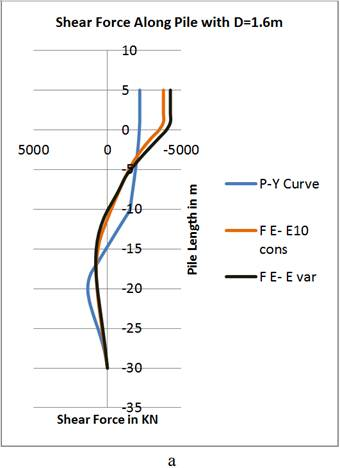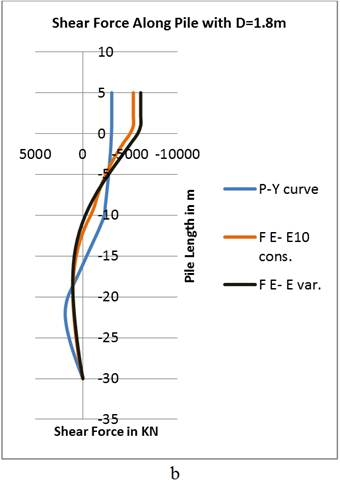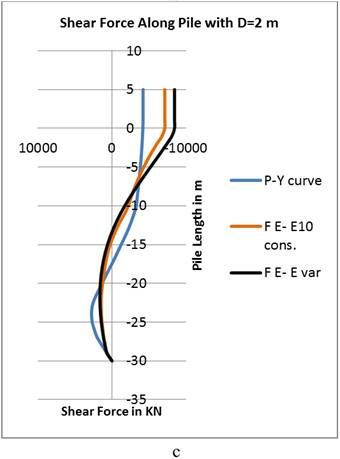Figure 3. The variation of shear force along the pile depth. (a- 1.6m diameter piles, b- 1.8m diameter piles and c- 2m diameter piles).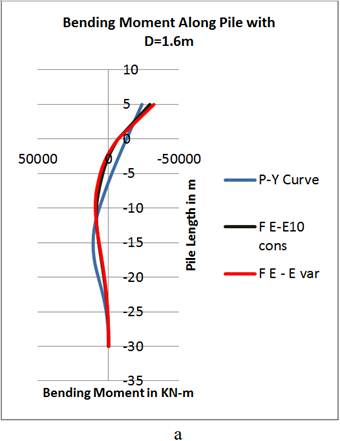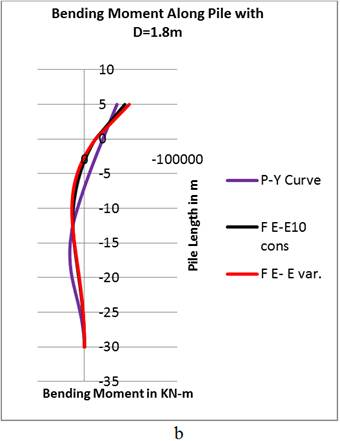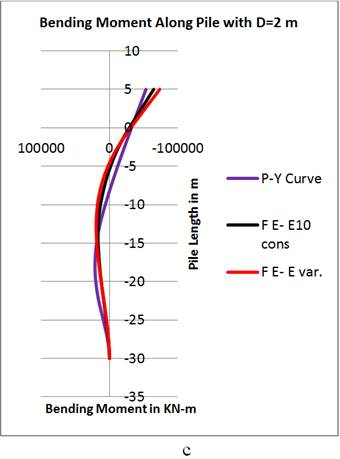Figure 4. The variation of bending moment along the depth of pile. (a- 1.6m diameter piles, b- 1.8m diameter piles and c- 2m diameter piles).

5. Conclusions and Recommendations

The following conclusions can be drawn from the cases studied:

(a).  Minimum ultimate lateral load capacity of piles is obtained from the P-Y curve method, and it is about half the values from the finite element methods.

(b).  There is a difference in shear force and bending moment distribution along the depth of the pile between the P-Y curve method and the finite element method which requires a good judgment in the evaluation and distribution of the steel reinforcement in the piles.

(c).  Increasing the rigidity of the piles lowers the location of the maximum shear force and bending moment in the piles in direct proportion to the rigidity.

To get better understanding of the behavior of the piles to the lateral loads, it is recommended to represent the soil by elsto-plastic nonlinear finite elements with the inclusion of a gap element to represent the bond between the pile and the soil.

References

1. Anderson, J., Townsend, F., and Grajales, B., 2003. Case history evaluation of laterally loaded piles. Journal of Geotechnical Geoenvironmental Engineering, ASCE, 129, 187-196.
2. Banerjee, P. K. and Davis, T. G., 1978. The behavior of axially and laterally loaded single piles embedded in nomohomogeneous soils. Geotechnique, 28, No. 3, 309-326.
3. Basu, D., and Salgado, R., 2007. Elastic analysis of laterally loaded pile in multilayered soil. Geomechanics Geoengineering, 2, 183-196.
4. Basu, D., and Salgado, R., 2008. Analysis of laterally loaded piles with rectangular cross sections embedded in layered soil medial. International Journal for Numerical Analysis Methods in Geomechanics. 32, 721-744.
5. Bransby, M. F., 1999. Selection of p-y curves for the design of single laterally loaded piles. International Journal for Numerical Analysis Methods in Geomechanics, 23, 1909-1926.
6. Brinch Hansen, J., 1961. The ultimate resistance of rigid piles against transversal forces. Bulletin no. 12. Danish Geotechnical Institute, Copenhagen, Denmark 5-9.
7. Broms, B., 1964. Lateral Resistance of Piles in Cohesiveness soils. Journal of Soil Mechanics and Foundation Division 90 (4), 27-63.
8. Brown, D. A., Shie, C. and Kumar, M., 1989. P-y curves for laterally loaded piles derived from three dimensional finite element model. Numerical Models in Geomechanics, NUMOG III, 683-690 (Elisevier Applied Science).
9. Brown, D.A., Shie, C.F., 1991. Some numerical experiments with a three-dimensional finite element model of laterally loaded piles. Computer and Geotechnics 12, 149-162.
10. Budhu, M. and Davis, T. G., 1988. Analysis of laterally loaded piles in soft clays. Journal of Geotechnical Engineering Division. ASCE, 114, 21-39.
11. Desai, C.S. and Appel G. C., 1976. 3-D analysis of laterally loaded structures. Numerical Methods in Geomechanics, Vol. 2, 405-418.
12. Dewaikar, D. M., Salimath, R. S. and Swant, V. A.,2009. A modified p-y curve for the analysis of laterally loaded pile in stiff clay. Australian Geotechnics Journal volume 44 No. 3, 91-99.
13. Guo, W. D. and Lee, F. H., 2001. Load transfer approach for laterally loaded piles. International Journal for Numerical Analysis Methods in Geomechanics, 25, 1101-1129.
14. Jeong, S.S., Seo, D.H., 2004. Analysis of tieback walls using proposed p-y curves for coupled soil springs. Computer and Geotechnics. 31, 443-456.
15. Jeremic, B., Yang, Z., 2002. Numerical analysis of pile behavior under lateral loads in layered elastic-plastic soils. International Journal for Numerical and Analytical Methods in Geotechnics 26, 1385-1406.
16. Klar, A. and Frydman, S., 2002. Three-dimensional analysis of lateral pile response using two-dimensional explicit numerical scheme. Journal of Geotechnical Geoenvironmental Engineering. ASCE, 128, 775-784.
17. Langer, A., Mosley, E. T., and Thompson, C., 1984. Laterally loaded deep foundations: Analysis and performance. ASTM STP 835, 239-243.
18. Lee, S. L., Kog, Y. C. and Karunaratne, G. P., 1987. Laterally loaded piles in layered soil. Soils and Foundations, 27, 1-10.
19. Matlock, H., 1970. Correlations for design of laterally loaded piles in clay. Paper no. OTC 1204, in: Proceedings of Second Annual Offshore Technology Conference, Houston, Texas, Vol. 1, 577-594.
20. Ng, C. W. W. and Zhang, L. M., 2001. Three-dimensional analysis performance of laterally loaded sleeved piles in sloping ground. Journal of Geotechnical Geoenvironmental Engineering, ASCE, 127, 499-509.
21. O'Neill, M.W., Gazioglu, S.M., 1984. Evaluation of p-y relationships in cohesive soils. Proceedings of a Analysis and Design of Pile Foundations, Geotechnical Engineering Division, 192-213.
22. Poulos, G. and Davis, H., 1980. Pile Foundation Analysis and Design, John Wiely & sons, Inc., New York.
23. Poulos, H. G., 1971a. Behavior of laterally loaded piles: I- single piles. Journal of Solid Mechanics, Foundation Division, ASCE, 97, 711-731.
24. Poulos, H. G., 1971b. Behavior of laterally loaded piles: III- socketed piles. Journal of Solid Mechanics, Foundation Division, ASCE, 98, 341-360.
25. Pyke, R. and Beikae, M., 1984. A new solution for the resistance of single piles to lateral loading. Laterally Loaded Deep Foundations, ASTM STP 835, 3-20.
26. Randolph, M. F., 1981. The response of flexible piles to lateral loading. Geotechnique, 31, No.2, 247-259.
27. Reese, C. and Matlock, H., 2956. Non-dimensional solutions for laterally loaded piles with soil modulus assumed proportional to depth. The 8th Texas Conference on Soil Mechanics and Foundation Engineering. Austin, 1-41.
28. Reese, L. C. and Cox, W. R., 1969. Soil behavior from analysis of tests of uninstrumented piles under lateral loading. Performance of Deep Foundations, ASTM STP 444, 160-176.
29. Reese, L. C. and Van Impe, W. F., 2001. Single pile and pile groups under lateral loading (A. A. Balkema: Rotterdam, Netherland).
30. Reese, L. C., Cox, W. R. and Koop, F. D., 1974. Analysis of laterally loaded piles in sand. Proceedings of 6th Offshore Technical Conference, Houston, Texas, volume 2, 473-483.
31. Reese, L. C., Cox, W. R. and Koop, F. D., 1975. Field testing and analysis of laterally loaded piles in stiff clay. Proceedings of 7th Offshore Technical Conference, Houston, Texas, volume 2, 671-690.
32. Reese, L.C., 1983. Behavior of piles and pile groups under lateral loads. Rep. to the U.S. Dept. of Transportation. Federal Highway Administration, Office of Research, Development, and Technology, Washington, D.C.
33. Robert, D. Cook, 1995. Finite Element Modeling for Stress Analysis, 1st Ed. USA: John Wiely and Sons.
34. Sun, K., 1994. Laterally loaded piles in elastic media. Journal of Geotechnical Engineering, ASCE, 120, 1324-1344.
35. Tak Kim, B., Kim, N., Jin Lee, W., and Su Kim, Y., 2004. Experimental load-transfer curves of laterally loaded piles in Nak-Dong River sand. Journal of Geotechnical Geoenvironmental Engineering, ASCE, 130, 416-425.
36. Trochanis, A. M., Bielak, J. and Christiano, P., 1991. Three-dimensional nonlinear study of piles. Journal of Geotechnical Engineering, ASCE, 117, 429-447.
37. USACE, 1998. Design of Deep Foundations: Technical Notes. US Army Corps of Engineers, Engineering Research and Development Center, Washington DC.
38. Verruijt, A. and Kooijman, A. P., 1989. Laterally loaded piles in layered elastic medium. Geotechnique, 39, No. 1,39-46.
39. Wallace, J. W., Tehrani, P. K., Ahlberg, E.R., Stewart, J. P., Taciroglu, E., 2014. Nonlinear load-deflection behavior of reinforced concrete drilled piles in stiff clay. Journal of Geotechnical Geoenvironmental Engineering, ASCE, 140, 1-14.
40. Won, J. O., You, K. H., Jeong, S. S., Kim, S. i., 2005. Coupled effects in stability analysis of pile slope systems. Computer and Geotechnics 32, 304-315.

 Contents 1. 2. 3. 3.1. 3.2. 4. 4.1. 4.2. 4.3. 4.4. 5.
Article ToolsAbstractPDF(1551K)